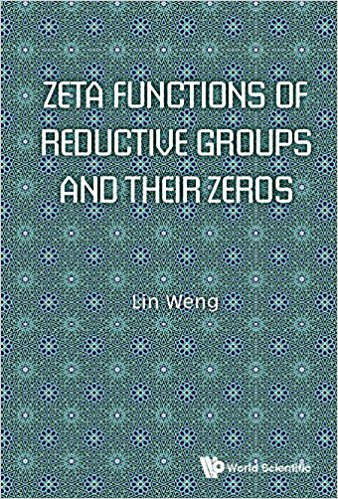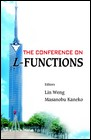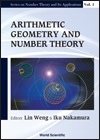# Lin WENGProfessor of Mathematics Affiliation: Graduate School of Mathematics Kyushu University Japan Office: W1-C627, Kyushu University Motooka 744, Fukuoka 819-0395 Personal data: Born in Dec. 1964, China Ph.D, Univ. of Sci. and Tech. of China, 1988 Office hour: Any time when in office Phone: (092) 802-4478 (092) 802-4478 (fax) E-Mail (the best way to reach me): weng@math.kyushu-u.ac.jp Web address: http://www$\small{\color{Red}{2}}$.math.kyushu-u.ac.jp/~weng/

### Biographical sketch

Lin WENG studied and worked on classical algebaric geometry in 1980's in China. During his 1989-1991 stay at Max-Planck Institute for Mathematics, he shifted his interests to arithmetic geometry. Then, Weng worked on arithmetic Grothendieck-Riemann-Roch theorem, by introducing relative Bott-Chern secondary characteristic classes. He moved to Osaka University in 1997. At the end of 1990's, he applied Arakelov geometry to moduli spaces of punctured Riemann surfaces, existence of Kaehler-Einstein metrics and stability. Since 1999, Weng has focused on the Program on Geometric Arithmetic, by completing a non-abelian class field theory for function fields over complex numbers, establishing a global cohomology theory for number fields, introducing non-abelian zeta functions and discovering the Weng zeta functions for (reductive group, maximal parabolic subgroup)s for global fields. Before transfering to Kyushu University in 2002, Weng also worked at Kobe University and Nagoya University. His current interests include Algebraic/Arithmetic/Complex Geometry and Number Theory. His very recent book on Zeta Functions of Reductive Groups and Their Zeros published in 2018 by the World Scientific.

### Books written and editedZeta Functions of Reductive Groups and Their Zeros Written by L. Weng w/ 5 Appendices joint w/ K. Sugahara on ARITHMETIC ADELIC COHOMOLOGY World Scientific, 2018 This book provides a systematic account of several breakthroughs in the modern theory of zeta functions. It contains two different approaches to introduce and study genuine zeta functions for reductive groups (and their maximal parabolic subgroups) defined over number fields. Namely, the geometric one, built up from stability of principal lattices and an arithmetic cohomology theory, and the analytic one, from Langlands' theory of Eisenstein systems and some techniques used in trace formula, respectively. Apparently different, they are unified via a Lafforgue type relation between Arthur's analytic truncations and parabolic reductions of Harder-Narasimhan and Atiyah-Bott. Dominated by the stability condition and/ or the Lie structures embedded in, these zeta functions have a standard form of the functional equation, admit much more refined symmetric structures, and most surprisingly, satisfy a weak Riemann hypothesis. In addition, two levels of the distributions for their zeros are exposed, i.e. a classical one giving the Dirac symbol, and a secondary one conjecturally related to GUE. This book is written not only for experts, but for graduate students as well. For example, it offers a summary of basic theories on Eisenstein series and stability of lattices and arithmetic principal torsors. The second part on rank two zeta functions can be used as an introduction course, containing a Siegel type treatment of cusps and fundamental domains, and an elementary approach to the trace formula involved. Being in the junctions of several branches and advanced topics of mathematics, these works are very complicated, the results are fundamental, and the theory exposes a fertile area for further research.Algebraic and Arithmetic Structures of Moduli Spacesedited by I. Nakamura & L. Weng Advanced Studies in Pure Mathematics, 58 Math Soc. Japan, 2010 The conference "Algebraic and Arithmetic Structures of Moduli Spaces" was held in September 2007, at Sapporo (Hokkaido university). Twenty talks were delivered by invited speakers on arithmetic geometry, algebraic geometry and complex geometry. This volume is the proceedings of the conference, to be exact, a collection of eleven papers contributed by some of the speakers which have undergone rigorous refereeing. The topics that are discussed in the articles are diverse in nature such as class field theory, zeta functions, moduli of arithmetic vector bundles, moduli of complex vector bundles, moduli of abelian varieties and theory of display, moduli of Fermat varieties and some topics on cubic threefolds. Among others, the papers of Pappas-Rapoport, Rajan and Weng address many new interesting questions in the related fields, which seem to be worthy of reading for young researchers.Conference on $\small{L}$-Functionsedited by L. Weng & M. Kaneko World Scientific 2007 This invaluable volume collects papers written by many of the world's top experts on L-functions. It not only covers a wide range of topics from algebraic and analytic number theories, automorphic forms, to geometry and mathematical physics, but also treats the theory as a whole. The contributions reflect the latest, most advanced and most important aspects of L-functions. In particular, it contains Hida's lecture notes at the conference and at the Eigenvariety semester in Harvard University and Weng's detailed account of his works on high rank zeta functions and non-abelian L-functions.Arithmetic Geometry and Number Theoryedited by L. Weng & I. Nakamura World Scientific, 2006 Mathematics is very much a part of our culture; and this invaluable collection serves the purpose of developing the branches involved, popularizing the existing theories and guiding our future explorations. More precisely, the goal is to bring the reader to the frontier of current developments in arithmetic geometry and number theory through the works of Deninger-Werner in vector bundles on curves over p-adic fields; of Jiang on local gamma factors in automorphic representations; of Weng on Deligne pairings and Takhtajan-Zograf metrics; of Yoshida on CM-periods; of Yu on transcendence of special values of zetas over finite fields. In addition, the lecture notes presented by Weng at the University of Toronto from October to November 2005 explain basic ideas and the reasons (not just the language and conclusions) behind Langlands' fundamental, yet notably difficult, works on the Eisenstein series and spectral decompositions. And finally, a brand new concept by Weng called the Geometric Arithmetic program that uses algebraic and/or analytic methods, based on geometric considerations, to develop the promising and yet to be cultivated land of global arithmetic that includes non-abelian Class Field Theory, Riemann Hypothesis and non-abelian Zeta and L Functions, etc.Hyperbolic Metrics, Selberg Zeta Functions and Arakelov Theory for Punctured Riemann Surfaces Lin WENG Lecture Note Series in Mathematics Osaka University 6(1998) Studies the Arakelov aspects of the moduli spaces of punctured Riemann surfaces, using hyperbolic metrics. Many fundamental metrized line bundles are introduced and applied to topics such as the arithmetic Riemann-Roch theorem for (singular) hyperbolic metrics, the local index theorem discovered by Tanktajan-Zograf through second variations of Selberg zeta functions, the Weil-Petersson metric and Tanktajan-Zograf metrics on the moduli spaces of punctured Riemann surfaces. Lecture notes given at Osaka University in 1997-1998Relative Bott-Chern Secondary Characteristic Classes and Arithmetic Grothendieck-Riemann-Roch Theorem I, II Lin WENG Preprints of Max-Planck Institute for Mathematics, 4732(91-79), 4986(94-51), 4987(94-52)(1991, 1994, 1994), pp.24, 261, 206 In these two volumes (and an original short announcement), we introduce six axioms for relative Bott-Chern secondary characteristic classes and prove the uniqueness and existence theorem for them. Such a work provides us a natural way to understand and hence to prove the arithmetic Grothendieck-Riemann-Roch theorem for l.c.i. morphisms which are smooth at infinity. These works were written earlier in 1997 mainly, and have never been published.
 © 2011-Now Lin WENG Last update: October 28, 2012 10:00 AM